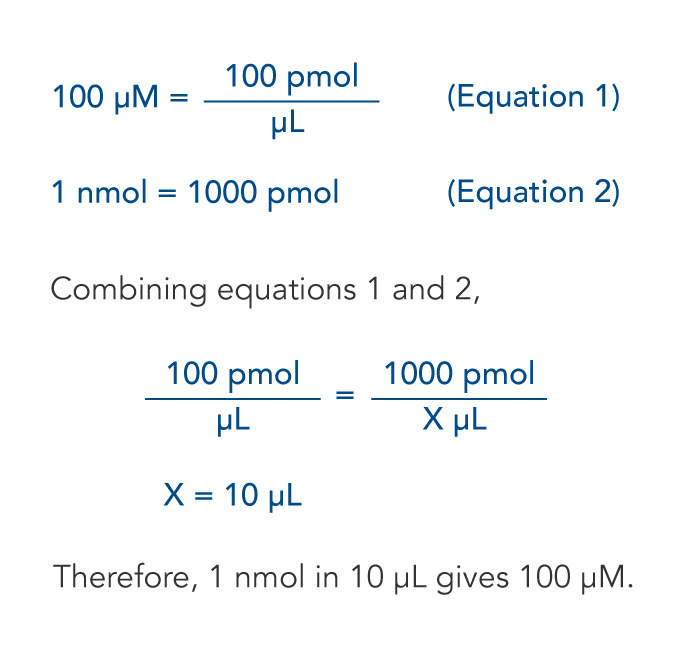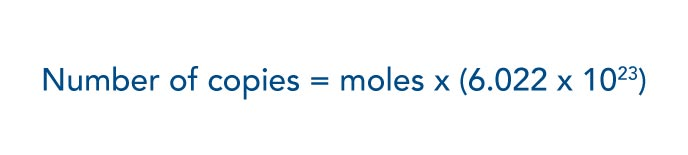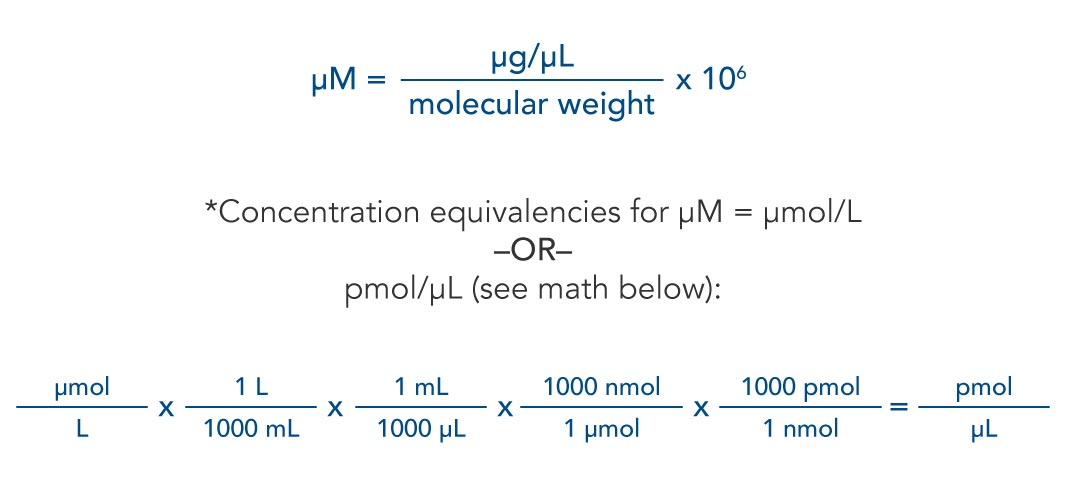# Calculation tips for resuspending and diluting nucleic acidsLab tips: Use these simple guidelines for making a 100 µM solution; calculating nanomoles, micrograms, copy number, and concentration; and determining concentration equivalencies.

Below are some of the common calculations we use when we work with oligonucleotides in our labs. You can also use the free, online IDT Resuspension Calculator to make most of these calculations.

### Making a 100 μM solutionTo resuspend your oligonucleotides to 100 μM, simply multiply the number of nanomoles (nmol) by 10 to get the volume (in μL) of water or buffer to add. The equation on the left shows an example, assuming you have an oligonucleotide of 1 nmol final yield.

### Calculating nmolUse this formula to calculate nmol when only the OD (absorbance at 260 nm) and extinction coefficient are provided.

### Calculating microgramsUse this formula to calculate micrograms (μg) of oligo when nmol and molecular weight (g/mol) are provided.

### Calculating copy numberUse this formula to calculate the number of copies of your DNA sequence when moles are provided. Note that 6.022 x1023 is Avogadro's number, the number of molecules in 1 mole.

### Calculating concentrationUse these formulas to calculate molar concentration (μM) when molecular weight (g/mol) and concentration in μg/μL are provided.

Access the free, online IDT Resuspension Calculator.

Published Nov 4, 2012
Revised/updated Mar 21, 2018